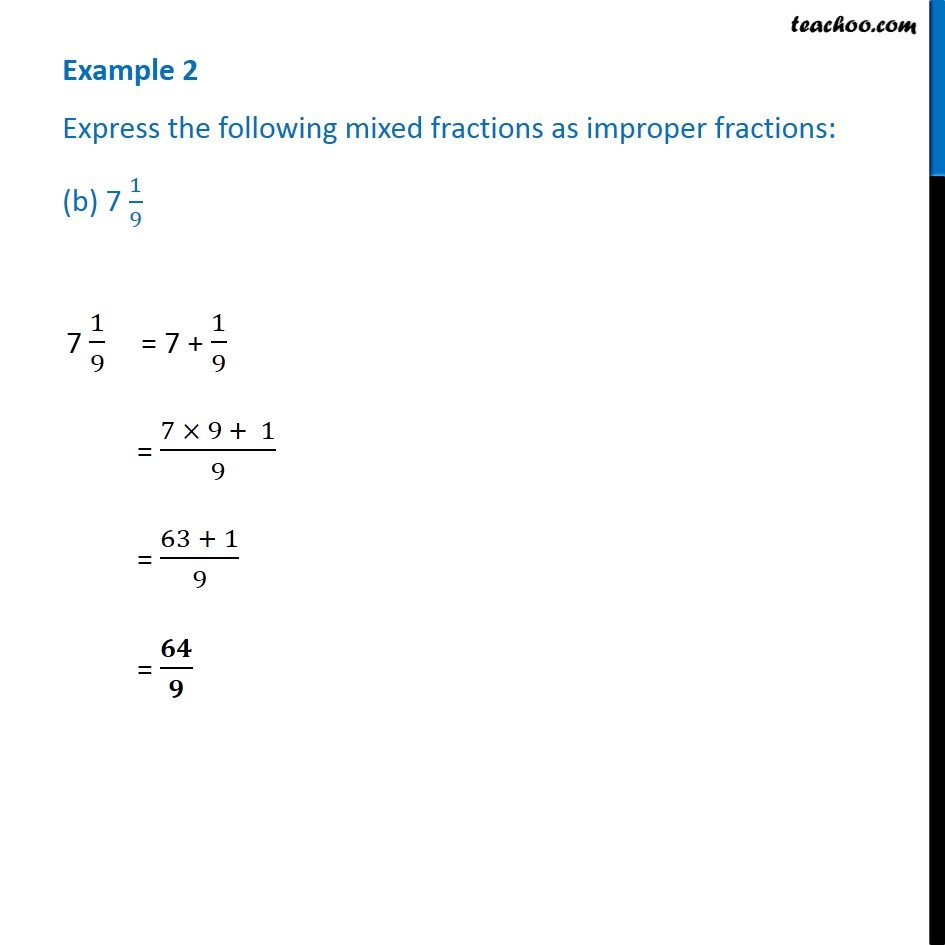Examples

Chapter 7 Class 6 Fractions
Serial order wiseLearn in your speed, with individual attention - Teachoo Maths 1-on-1 Class

### Transcript

Example 2 Express the following mixed fractions as improper fractions: (b) 7 1/9 7 1/9 = 7 + 1/9 = (7 × 9 + 1)/9 = (63 + 1)/9 = 𝟔𝟒/𝟗